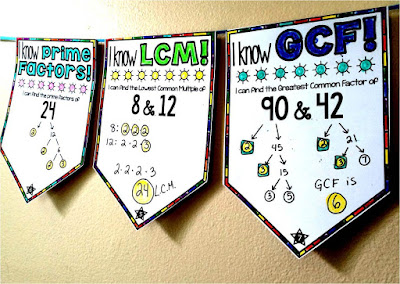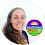## Pages

### Finding GCF and LCM with the Ladder (or Cake) Method

When I first came across the ladder method (ie: the upside-down cake method) for finding greatest common factors and lowest common multiples, I thought it was nothing short of complete genius. This was pretty recent, too! I love learning new methods for teaching math concepts.

Prime factoring is super cool and extremely useful in building number sense, but if the goal is to find GCF and especially LCM, this cake method makes the process so much easier.

Here is a simple video explaining the process:

On the outside of the upside down cake are all the factors the two numbers have in common. On the bottom are the "leftover" numbers.

The GCF is the product of all the common factors on the left. The LCM is the product of the GCF and the "leftovers" on the bottom.I wanted to make a math word wall reference for this method that students could refer to whenever they are finding GCF, LCM or even simplifying fractions (another neat use for this method brought up in our Visual Math Facebook group). You can get the GCF & LCM word wall references free in my math resource library. This math word wall reference is also part of my 6th grade math word wall.

For practice, I made this set of self-checking GCF & LCM solve 'n check task cards. Students find GCF and LCM on the card then add, subtract, multiply or divide their answers and compare against the check number. Changes are if the numbers match, GCF and LCM were found correctly.

And a new
GCF and LCM digital math escape room.

Another cool discussion that came up in the FB group was about relative prime numbers. In the word wall reference above, LCM= 2x2x2x3x10. While 10 is not prime, it is relatively prime because it and 3 have no more factors in common.

So we have the cake method for finding GCF and LCM and we have good old prime factoring. Any other methods?

When I was in grad school we learned about the Euclidean algorithm for finding GCF. Above is an example of how this algorithm works for finding that the GCF of 81 and 57 is 3.

I still like the cake method better :)I made this GCF, LCM and Prime Factors math pennant before learning about the cake method. It was that recent! I think using the cake method would be a great way to complete the problems on the pennants.

You may say I'm a touch obsessed with prime numbers. We learned how they are used in PIN codes and other super cool spy stuff in grad school (though I only remember shadows of all this now).

What has stuck with me is that every number has its own, unique "prime number fingerprint". No two numbers share the same string of factors. Super cool.

It seems so obvious now, but when I realized this, numbers started feeling a lot like building blocks, almost like chemical elements.

A few months ago I added this prime factorization reference to my 4th grade math word wall. I also pulled these pieces out for my blog (you can get them as part of my free math resource library).How do you teach finding GCF and LCM? Do you have kids prime factor or do you use the cake method? Something else?

Activities for Teaching GCF and LCM

Digital Math Escape Rooms

Math Word WallsFree math resource library

1.The cake method is a cool way, however it could become problematic if we try to use it to find LCM for 3 (or more) numbers. Example: LCM of 8, 12, 16 - the cake method will show GCF of 4 with remaining 2, 3, 4 and student will think the LCM is 4x2x3x4=96, but in reality the LCM is 48. Because of that, the prime factorization/factor tree might be safer method. But the cake method will be good if only work with 2 numbers for GCF & LCM. :D

1.Yes I came to say the same thing. I only use the cake method for GCF because it works when you need the GCF of 3 numbers but for LCM they need an alternative method anyway so I stick with listing the multiples on the M.

2.UNLESS you're like me. I just found out the proper way to use the CAKE METHOD. If you are using 3 numbers-it still works. For example, the LCM of 8,12, 16. I started with dividing them all by 4 which gave me the remainders of 2, 3, 4. YOU CAN DIVIDE ONLY TWO OF THE THREE-WHICH MEANS I CAN DIVIDE THE 2 AND 4, NOT THE 3. IF YOU CAN'T DIVIDE IT, YOU JUST BRING IT DOWN AS A REMAINDER. MIND BLOWN. SO once you do that, you end up with 1, 3 and 2 in your final layer. THEN making the shape of an L would give you 4x2x1x3x2=48. IT DOES WORK EVERYTIME! Hope this helps!

2.It works if you do it in multiple steps - first using 8 and 12 to get an LCM of 24. Then using 24 and 16 to get an LCM of 48. Or you could start with 8 and 16 (16) and then do 16 and 12 to get 48. Or start with 12 and 16 (48) and then do 12 and 48 (still 48).

1.Alternatively, you can do all 3 numbers at once first factoring out everything possible from all 3 numbers, then factoring out anything from 2 numbers and just dropping down the number you didn't divide. In this case after dividing 4 you then chose 2 and get 1, 3, 2 remaining on the bottom. The LCM is 4*2*1*3*2=48 which is the actual LCM. You stop at 4 for the GCF though, that's only the things taken out of all 3 numbers multiplied together. I love this method, it's so amazing!

3.My concern with this method is that it doesn't move them toward algebra readiness. We switch to the prime factorization method so they are ready to apply it to finsing the GCF and LCM of polynomials in their study of rational expressions and equations. Are we going to start teaching the cake method for polynomials also? Otherwise with this easy pass right now, we're setting them up for failure later on.

1.Valid concern! The cake method can be used for polynomials, too. I think there's value in showing students that there are multiple ways to approach math problems and that part of their job as students is to find the method that works best for them :)

Comment